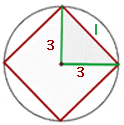The circumference is the length around a circle, that is to say, it is the perimeter of the circle.If the circumference of a circle is 43.96 cm, what is its area?Calculate the circumference of a wheel with a diameter of 90 cm.Calculate the area of the square inscribed in a circle with a circumference of 18.84 cm.By applying the Pythagorean theorem for one of the triangles, we obtain:Did you like the article?(1 votes, average: 5.00 out of 5)Loading...

Emma

I am passionate about travelling and currently live and work in Paris. I like to spend my time reading, gardening, running, learning languages and exploring new places.

Did you like
this resource?

Bravo!